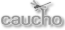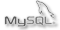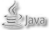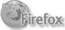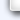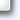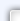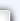User: alciroOriginal    Translate to:  Deutsch  English  Français  中文## Microcontroladores 8051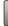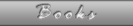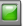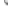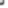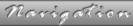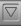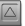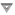1. 8051 Microcontrollers2. Memory structure in the 8051 microcontrollers3. 8051 addressing modes4. Types of instructions in the microcontroller 80515. Assembler Programming6. Programming in C7. Serial communications10. Reference Guide 8051 microcontrollers## 6.3. Bit field structures

You can define the elements of a structure bit fields acting as member variables of structure, with a number of bits between 1 and 8 (the range of structures depends on the bit microprocessor architecture and compiler being using, the range can be from 1 to 8, 1 to 16, 1 to 32 or 1 to 64).

Declaration of structure data type bits

``` / / Declaring a bit structure
Ports struct {
CH0 unsigned: 1, / / Range 0.1
Ch1 unsigned: 2; / / range 0 to 3
Ch2 unsigned: 3; / / range 0 to 7
unsigned Ch3: 1, / / Range 0.1
}; ```

Declaration of variables

``` void main (void) {
struct data ports PU_1 / / Declaring a variable rate PU_1 Ports

PU_1.Ch0 = 0, / / Assign data items
PU_1.Ch1 = 3;
PU_1.Ch2 = 5;
PU_1.Ch3 = 1;
... ```

Memory contents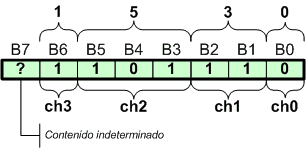Memory contents of the bit field structure

## 6.4. Unions

Data type declaration

``` {Data junction
unsigned int i; / / Integer
unsigned char c  / / Array of two characters
} ```

Declaration of variables

``` void main (void) {
Data junction d_1 data / / Declaration of a variable data rate d_1
d_1.i = 0x0405, / / ​​Assignment of an integer number
d_1.c ch1 =  / / Assignment to ch1 1 byte contents of the union (ch1 = 5)
CH2 = d_1.c  / / Assignment to ch1 1 byte contents of the union (CH2 = 4) ```

Memory contents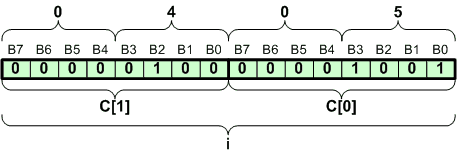Memory contents of the union

## 6.5. Definition of symbols

Definition of symbols

``` {# Define principle
# Define end}
# Define integer int
# Define number 10
# Define limited number * 5
# Define sum x + y
# Define message "other large \ n" ```
``` main ()
principle
integer x, y, z;
x = 5;
y = limit;
z = sum;
if (z> y)
printf (message);
...
order ```

Loading
copyright © 2007-2021  www.alciro.org  All rights reserved.